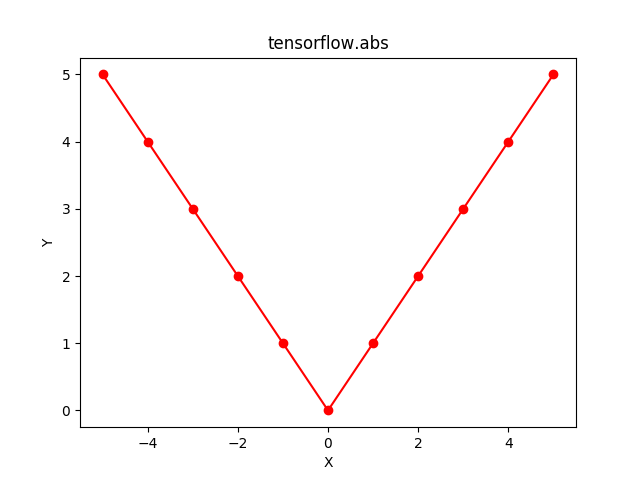# Python | Tensorflow abs() method

Tensorflow is an open-source machine learning library developed by Google. One of its applications is to develop deep neural networks.

The module tensorflow.math provides support for many basic mathematical operations. Function tf.abs() [alias tf.math.abs] provides support for the absolute function in Tensorflow. It expects the input in form of complex numbers asor floating point numbers. The input type is tensor and if the input contains more than one element, an element-wise absolute value is computed.

For a complex number, the absolute value is computed as.
For floating point numbers, the absolute value is computed asSyntax: tf.abs(x, name=None) or tf.math.abs(x, name=None)

Parameters:
x: A Tensor or SparseTensor of type float16, float32, float64, int32, int64, complex64 or complex128.
name (optional): The name for the operation.

Return type: A Tensor or SparseTensor with the same size and type as that of x with absolute values. For complex64 or complex128 input, the returned Tensor will be of type float32 or float64, respectively.

Code #1: For Floating point numbers

 # Importing the Tensorflow library  import tensorflow as tf     # A constant vector of size 5  a = tf.constant([-0.5, -0.1, 0, 0.1, 0.5], dtype = tf.float32)     # Applying the abs function and  # storing the result in 'b'  b = tf.abs(a, name ='abs')     # Initiating a Tensorflow session  with tf.Session() as sess:      print('Input type:', a)      print('Input:', sess.run(a))      print('Return type:', b)      print('Output:', sess.run(b))

Output:

Input type: Tensor("Const:0", shape=(5, ), dtype=float32)
Input : [-0.5 -0.1  0.   0.1  0.5]
Return Type : Tensor("abs:0", shape=(5, ), dtype=float32)
Output : [0.5 0.1 0.  0.1 0.5]


Code #2: Visualization

 # Importing the Tensorflow library  import tensorflow as tf     # Importing the NumPy library  import numpy as np     # Importing the matplotlib.pylot function  import matplotlib.pyplot as plt     # A vector of size 11 with values from -5 to 5  a = np.linspace(-5, 5, 11)     # Applying the absolute function and  # storing the result in 'b'  b = tf.abs(a, name ='abs')     # Initiating a Tensorflow session  with tf.Session() as sess:      print('Input:', a)      print('Output:', sess.run(b))      plt.plot(a, sess.run(b), color = 'red', marker = "o")       plt.title("tensorflow.abs")       plt.xlabel("X")       plt.ylabel("Y")          plt.show()

Output:

Input: [-5. -4. -3. -2. -1.  0.  1.  2.  3.  4.  5.]
Output: [5. 4. 3. 2. 1. 0. 1. 2. 3. 4. 5.]Code #3: For Complex Numbers

 # Importing the Tensorflow library  import tensorflow as tf     # A constant vector of size 2  a = tf.constant([[-2.25 + 4.75j], [-3.25 + 5.75j]],                                dtype = tf.complex64)     # Applying the abs function and  # storing the result in 'b'  b = tf.abs(a, name ='abs')     # Initiating a Tensorflow session  with tf.Session() as sess:      print('Input type:', a)      print('Input:', sess.run(a))      print('Return type:', b)      print('Output:', sess.run(b))

Output:

Input type: Tensor("Const_1:0", shape=(2, 1), dtype=complex64)
Input : [[-2.25+4.75j] [-3.25+5.75j]]
Return Type : Tensor("abs_1:0", shape=(2, 1), dtype=float32)
Output : [[5.255949 ] [6.6049223]]


My Personal Notes arrow_drop_upCheck out this Author's contributed articles.

If you like GeeksforGeeks and would like to contribute, you can also write an article using contribute.geeksforgeeks.org or mail your article to contribute@geeksforgeeks.org. See your article appearing on the GeeksforGeeks main page and help other Geeks.

Please Improve this article if you find anything incorrect by clicking on the "Improve Article" button below.

Article Tags :

Be the First to upvote.

Please write to us at contribute@geeksforgeeks.org to report any issue with the above content.